5th Grade Science : Identify materials based on properties with measurement

Example Questions

Example Question #1 : Identifying Material Properties

You have two round objects that are both roughly the size of a basketball. One of them weighs 50 pounds, and the other weighs 2 pounds. One is made of rubber and one is made of iron. Which object is more likely to be made of iron?

the 50 pound object

the 2 pound object

not enough information

the 50 pound object

Explanation:

Iron has a higher density than rubber, meaning that it's weight or mass will be greater even if it's the same size. Standard units are used to measure and describe physical quantities such as weight, time, temperature, and volume.

Example Question #2 : Identifying Material Properties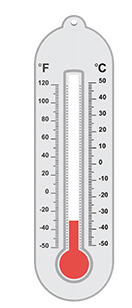You measure the temperature of a substance. The thermometer shows the results. How would you record the temperature of the substance?

50 degrees F

-50 degrees F

-30 degrees F

30 degrees F

-30 degrees F

Explanation:

The answer is "-30 degrees Fahrenheit"

Standard units are used to measure and describe physical quantities such as weight, time, temperature, and volume. The standard unit for temperature is degrees Fahrenheit. Volume is measured in Liters, weight in pounds, and time is measured in minutes, seconds, or hours.

Example Question #3 : Identifying Material Properties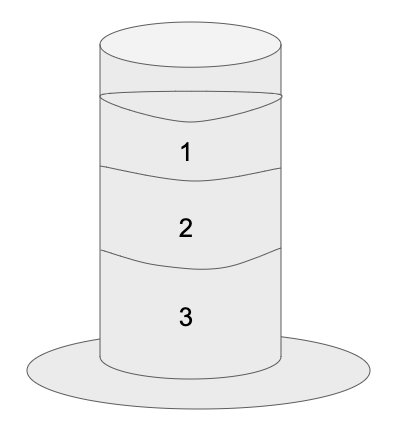There are three different liquids layered in the graduated cylinder pictured. If we know that vegetable oil is more dense than water but less dense than honey, which of the liquids is most likely vegetable oil?

liquid number 1

liquid number 2

not enough information

liquid number 3

liquid number 2

Explanation:

The answer is liquid number 2. Density is a measure of the amount of "stuff" in a given volume. More dense objects sink and less dense objects float.

Example Question #4 : Identifying Material Properties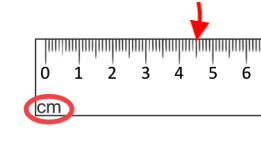How many millimeters are being measured at the point where the red arrow is shown?

45 mm

450 mm

40 mm

4.5 mm

45 mm

Explanation:

The answer is 45 because there are 10 millimeters in a centimeter and the ruler is measuring 4.5 centimeters.

Example Question #5 : Identifying Material Properties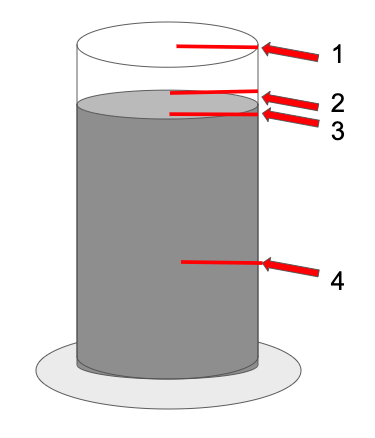At which number should the volume of this liquid be measured?

at number 2

at number 4

at number 3

at number 1

at number 3

Explanation:

The answer is "at number 3" because you measure volume at the meniscus.

Example Question #6 : Identifying Material Properties

Dev and Matt are comparing two samples of matter. They made a table of the properties of each sample.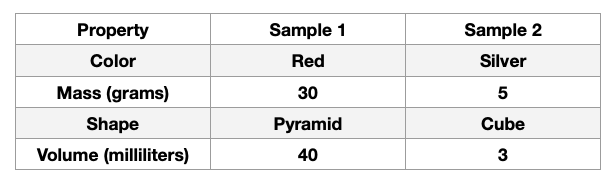Which property provides the best evidence that both samples are solids rather than liquids?

Shape

Volume

Mass

Color

Shape

Explanation:

This data table provides observations and measurements of two mystery samples. Properties of materials can be used to determine what objects are or the qualities of them. In this case, we are looking for the property that would allude to these samples being solids instead of liquids, and that property is the shape of the samples. Liquids or solids can be measured for mass and volume and described by color, but cannot be characterized by shape. Liquids take the form of the container that they are in, whereas solids maintain a specific shape. These samples are solids because they are a cube and pyramid.

Example Question #7 : Identifying Material Properties

Four students in a science class observed and collected data about the properties of a different material. The data table below shows the student observations.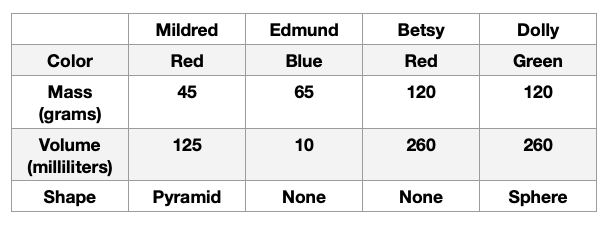Based on this data chart, which statement correctly describes one of the materials?

Dolly observed a liquid.

Edmund observed a solid.

Betsy observed a liquid.

Mildred observed a gas.

Betsy observed a liquid.

Explanation:

This data table lists what each student observed when they were looking at their material. Some of the properties that describe this material can be gathered through observation, while others are through measurement. In this case, what type of matter the student is looking at comes down to the shape. A gas, liquid, or solid can be any color, mass, or volume, so those characteristics are less helpful in identifying what these materials are. Gases have no shape, liquids take the form of the containers they are in, and solids have a definite figure. Mildred and Dolly look at solids because the material is described as a pyramid and a sphere, which are definite shapes. Edmund and Betsy look at materials with no shape, which means they are gas or liquid, so the only answer choice that matches is "Betsy observed a liquid.".

Example Question #8 : Identifying Material Properties

True or False: The physical properties of matter can only be gathered through measuring.

False

True

False

Explanation:

The statement "The physical properties of matter can only be gathered through measuring." is false. The physical properties of matter can be gathered through measurement AND observation. Using the five senses to collect information about the material or matter is just as crucial as taking concrete measures like the mass or volume.  Classification and categorization of material are best done through a combination of observation and measurement.

Example Question #9 : Identifying Material Properties

Which of the physical properties listed below can be measured?

Boiling point

Freezing point

All of the answer choices are correct.

None of the answer choices are correct.

Ability to conduct electricity

All of the answer choices are correct.

Explanation:

All of the physical properties listed below can be determined through measurement. A material's freezing point can be determined by keeping track of the temperature the material is exposed to colder and colder temperatures until it freezes. The temperature this happens is decided after multiple trials determine its freezing point. A material's boiling point is discovered by measuring a rising temperature until the material comes to a rolling boil. Both of these physical properties are measured with a thermometer. Finally, whether a material conducts electricity depends on what the sample is made up of. Things like wood, plastic, and cloth do not conduct electricity well, while metal and copper do. The electrical conductivity can be calculated by measuring the resistance, area, and length of the test material. You can also use a four-terminal ohmmeter for greater accuracy.

Example Question #10 : Identifying Material Properties

True or False: Texture is a physical property that is determined through measurement.

True

False